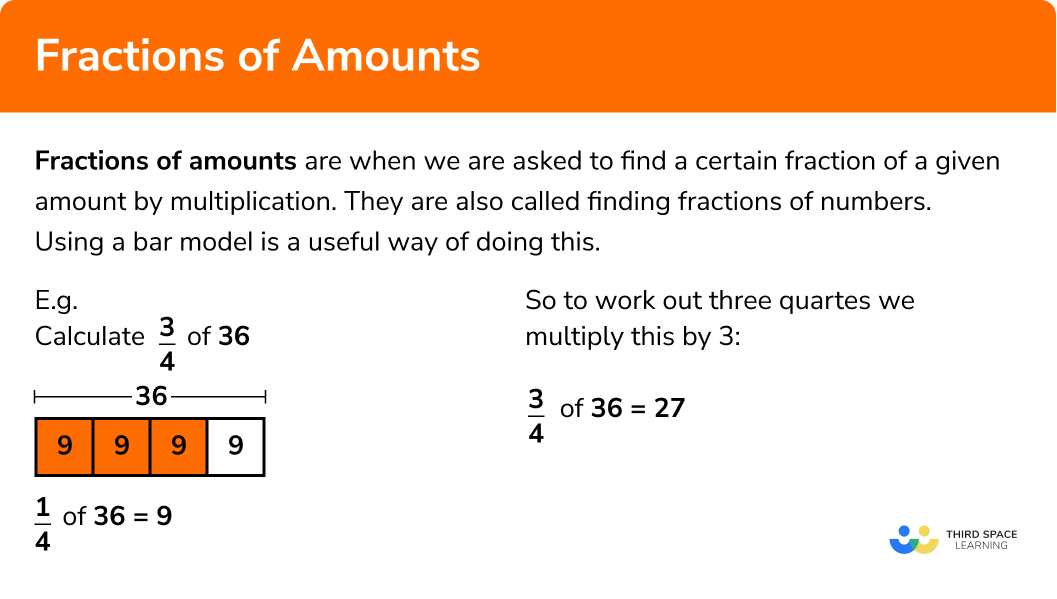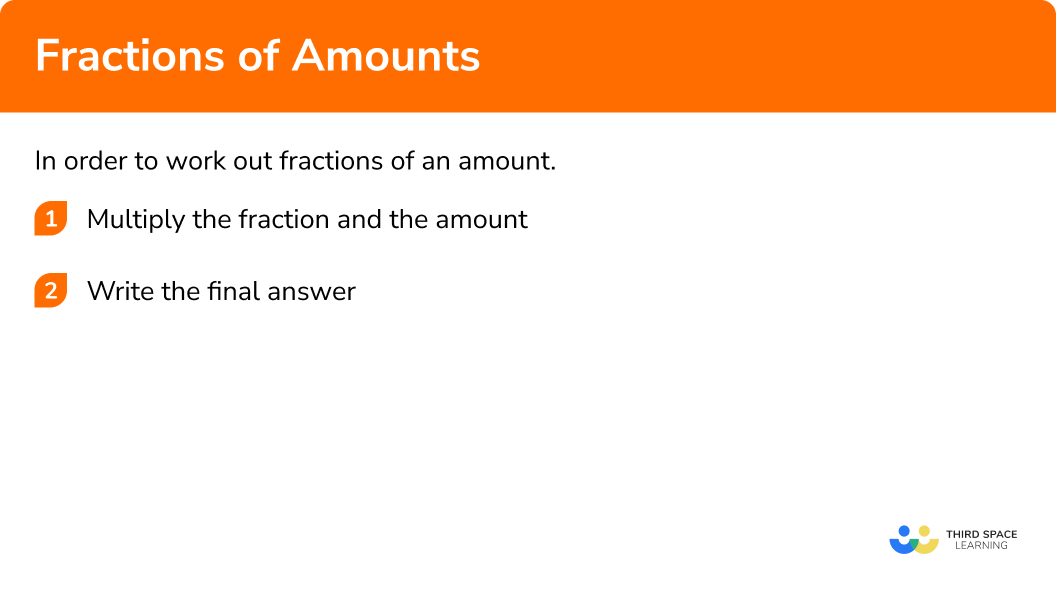GCSE Maths Number FDP Fractions

Fractions Of Amounts

# Fractions Of Amounts

Here we will learn about fractions of amounts.
There are also fractions worksheets, fraction activity sheets and fraction word problems (with answer sheets) based on Edexcel, AQA and OCR exam questions, along with further guidance on where to go next if you’re still stuck.

## What are fractions of amounts?

Fractions of amounts is the skill of finding a given fraction of a certain amount.

Example 1

E.g.

Find

$\frac{3}{4} \quad of \quad 36$

As we are asked to work out three quarters of 36, let’s start by working out one quarter:

$\frac{1}{4} \quad of \quad 36 = 9$

So to work out three quarters we multiply this by 3:

$\frac{3}{4} \quad of \quad 36 =27$

You can visualise this using a bar model.

Finding a fraction of a number is the same as multiplying a fraction by a number.

Example 2

\frac{2}{5} of 20 is the same as \frac{2}{5} \times 20

\frac{2}{5} of 20 = \frac{2}{5} \times 20 = \frac{2 \times 20}{5} = \frac{40}{5} = 8

We can find fractions of a quantity  including integers (whole numbers), decimals and fractions. When calculating a fraction of a quantity you can use either the method shown in E.g. 1 or E.g. 2 but sometimes one method will be easier to apply than the other.

Example 3

Find \frac{5}{6} of 0.4

\frac{5}{6} of 0.4 = \frac{5}{6} \times 0.4 = \frac{5 \times 0.4}{6} = \frac{2}{6} = \frac{1}{3}

### What are fractions of amounts?## One amount as a fraction of another

Sometimes it is helpful to express an amount of one thing as a fraction of the entire thing.

For example;

A shop sells rolls of ribbon. A full roll has 7 metres of ribbon on it. Sabastian buys 35 centimetres of ribbon. Express the length of Sabastian’s piece of ribbon as a fraction of a full roll of ribbon.

7 metres = 700 cm.

The fraction would therefore be \frac{35}{700}

This can simplify \frac{35 \div 7}{700 \div 7}=\frac{1}{20}

Therefore Sebastian has one twentieth of a full roll of ribbon.

Sometimes it is helpful to express a value as a fraction of another amount. This can then show the proportion of that value in comparison to the other amount.

For example,

Ivan has 3 pens in his school bag.

Jessica has 24 pens in her school bag.

Express the number of pens Ivan has as a fraction of the amount of pens Jessica has.

Ivan has 3 pens compared to Jessica’s 24 pens. As a fraction this is \frac{3}{24}

This can simplify

\frac{3 \div 3}{24 \div 3}=\frac{1}{8}

This fraction shows that Ivan has an eighth as many pens as Jessica.

## How to calculate fractions of amounts

In order to work out fractions of an amount:

1. Multiply the fraction and the amount.

### Explain how to work out fractions of amounts in 2 steps### Related lessons on fractions

Fractions of amounts is part of our series of lessons to support revision on fractions. You may find it helpful to start with the main fractions lesson for a summary of what to expect, or use the step by step guides below for further detail on individual topics. Other lessons in this series include:

## Fractions of amounts examples

### Example 1: unit fractions of amounts

Work out:

$\frac{1}{2} \quad of \quad 16$

1. Multiply the fraction and the amount.

The “of” means that we multiply the fraction and the amount.

$\frac{1}{2}\times16$

$\frac{1}{2}\times16=\frac{16}{2}=16\div2$

You divide the amount by the denominator (bottom number) of the fraction.

$\frac{1}{2}\times16=8$

### Example 2: unit fractions of amounts

Work out:

$\frac{1}{7} \quad of \quad 28$

The “of” means that we multiply the fraction and the amount.

$\frac{1}{7}\times28$
$\frac{1}{7}\times28=\frac{28}{7}=28\div7$

You divide the amount by the denominator (bottom number) of the fraction.

$\frac{1}{7}\times28=4$

### Example 3: non-unit fractions

Work out:

$\frac{3}{4} \quad of \quad 20$

The “of” means that we multiply the fraction and the amount.

$\frac{3}{4}\times20$
$\frac{3}{4}\times20=\frac{3\times20}{4}=15$

Alternatively, you could split the calculation into two parts.
First divide the amount by the denominator (bottom number) of the fraction to find one quarter of 20.
Then, multiply by the numerator (top number):

$\frac{1}{4} \quad of \quad 20=20\div4=5$

So,

$\frac{3}{4} \quad of \quad 20=3\times5=15$

$\frac{3}{4}\times20=15$

### Example 4: non-unit fractions

Work out:

$\frac{2}{7} \quad of \quad 21$

The “of” means that we multiply the fraction and the amount.

$\frac{2}{7}\times21$
$\frac{2}{7}\times21=\frac{2\times21}{7}=6$

Alternatively, you could split the calculation into two parts.
First divide the amount by the denominator (bottom number) of the fraction.
Then multiply by the numerator (top number):

$\frac{1}{7} \quad of \quad 21=21\div7=3$

So,

$\frac{2}{7} \quad of \quad 21=2\times3=6$

$\frac{2}{7}\times21=6$

### Example 5: calculator allowed

Work out:

$\frac{3}{5} \quad of \quad 62 kg$

The “of” means that we multiply the fraction and the amount.

$\frac{3}{5}\times62$
$\frac{3}{5}\times62=\frac{3\times62}{5}=37.2$

Alternatively, you could split the sum into two parts.
First divide the amount by the denominator (bottom number) of the fraction.
Then, multiply by the numerator (top number):

$\frac{1}{5}\quad of \quad62=62\div5=12.4$

So,

$\frac{3}{5}\quad of \quad62=62\times12.4=37.2$

$\frac{3}{5}\times62=37.2$

The final answer is 37.2 kg.

### Example 6: calculator allowed

Work out:

$\frac{7}{10}\quad of \quad135km$

The “of” means that we multiply the fraction and the amount.

$\frac{7}{10}\times135$

$\frac{7}{10}\times135=\frac{7\times135}{10}=94.5$

Alternatively,  you could split the sum into two parts.
First divide the amount by the denominator (bottom number) of the fraction.
Then multiply by the numerator (top number):

$\frac{1}{10}\quad of \quad135=135\div10=13.5$

So,

$\frac{7}{10}\quad of \quad135=13.5\times7=94.5$

$\frac{7}{10}\times135=94.5$

The final answer is 94.5 km.

### Common misconceptions

• Not writing money with two decimal places

If the answer to a question involving money is a decimal and the units are £ you need 2 digits after the decimal point for the pence.

E.g.

$\frac{2}{9} \quad of \quad£6.30$
$\frac{2}{9}\times6.3=1.4$

• Not fully answering the question

A common error is to

### Practice fractions of amounts questions

99.51.86\frac{1}{2}\times18=\frac{18}{2}=18\div2=9

156257\frac{1}{5}\times30=\frac{30}{5}=30\div5=6

2316820\frac{2}{3}\times24=\frac{2\times24}{3}=(24\div3)\times2=16

34402835\frac{5}{8}\times56=\frac{5\times56}{8}=(56\div8)\times5=35

2.1 kg4.5 kg6.3 kg5.9 kg\frac{3}{7}\times14.7=\frac{3\times14.7}{7}=(14.7\div7)\times3=6.3

1.6 km6.4 km3.2 km4.2 km\frac{4}{9}\times14.4=\frac{4\times14.4}{9}=(14.4\div9)\times4=6.4

### Fractions of amounts GCSE questions

1.   A jigsaw normally costs £9.60.How much does a jigsaw cost in the sale?

(2 marks)

\frac{1}{3}\times9.60=9.60\div3=3.2                                                                                                 (1)

9.6-3.2=6.4

The jigsaw costs £6.40 in the sale.

(1)

2. The average age of people in an office is 40 years old.

Sam’s age is \frac{11}{8} of the average.

How old is Sam?

(2 marks)

\frac{11}{8}\times40

(1)

Sam is 55.

(1)

3. A train has 1 first-class carriage and 4 standard carriages.

The first-class carriage has 40 seats.

\frac{7}{8} of the seats are being used.

Each standard class carriage has 60 seats.

\frac{5}{12} of the seats are being used.
Are more than half the seats on the train being used?

(5 marks)

40+(4\times60)=280

For working out the total number of seats on the train.

(1)

280\div2=140

For working out the half of seats on the train.

(1)

\frac{7}{8}\times40=35 \frac{5}{12}\times60=25

For working out the number of seats used the first-class carriage or second class carriage.

(1)

35+(4\times25)=135

For working out the total number of seats used on the train (1)

(1)

NO, only 135 seats are used

(1)

## Learning checklist

You have now learned how to:

• Find the fraction of an amount
• Find the fractions of quantities

## Still stuck?

Prepare your KS4 students for maths GCSEs success with Third Space Learning. Weekly online one to one GCSE maths revision lessons delivered by expert maths tutors.

Find out more about our GCSE maths tuition programme.